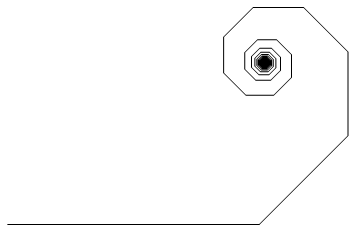# The Crazy Ant

Calculus Level 5A crazy ant is standing on the origin. It begins by walking 1 unit in the $+x$-direction and then turns $45^\circ$ counterclockwise and walks $\frac{1}{2}$ units in that direction. The ant then turns another $45^\circ$ and walks $\frac{1}{3}$ units in that direction. The ant keeps doing this endlessly. How far is the ant's final position from its initial position?

×